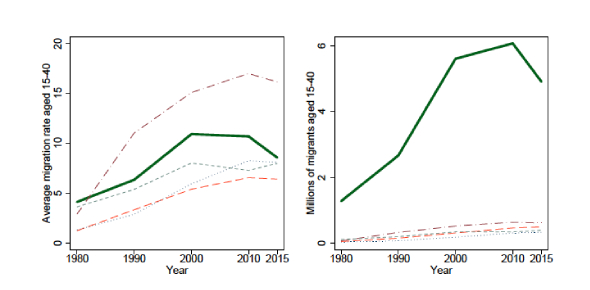# Population Growth And Migration: Quiz!

10 Questions | Total Attempts: 39Settings• 1.
What do demographic transition models show?
• A.

Rates of employment

• B.

Behavior changes over time

• C.

Population changes over time

• D.

Change in animal behaviour

• 2.
How is the birth rate measured?
• A.

The number of persons added to the population

• B.

The number of live births per 1000

• C.

The number of births per 1000

• D.

The number of persons living in urban areas

• 3.
How is population density measured?
• A.

Per kilometer square

• B.

Per kilometer

• C.

Per metre square

• D.

Per unit area

• 4.
High population density is also known as:
• A.

Densely populated

• B.

Sparsely populated

• C.

Unpopulated

• D.

Scarcely populated

• 5.
Population distribution is a term that refers to:
• A.

How population in an area changes over time

• B.

The number of people who die in relation to the number of people who live in a specific area

• C.

The way in which people are spread across a given area

• D.

The number of persons per square kilometer

• 6.
How is population density calculated?
• A.

Total population divided by total land area

• B.

Total land area divided by total population

• C.

Total population multiplied by total land area

• D.

Total population divided by 2

• 7.
Which of the following is not a short- term form of migration?
• A.

Sport

• B.

Medical

• C.

Retirement

• D.

Family vacation

• 8.
Moving to a new location to find employment is:
• A.

A social factor

• B.

An economic factor

• C.

A physical factor

• D.

An environmental factor

• 9.
What do we call a person who has been forced to leave their home, perhaps by war or a natural disaster (such as drought), and move away without having another home to go to?
• A.

An immigrant

• B.

A migrant

• C.

A refugee

• D.

• 10.
What do you call it when someone leaves a country to find work/live elsewhere?
• A.

Emigration

• B.

Immigration

• C.

Migration

• D.

Relocation

Related TopicsBack to top